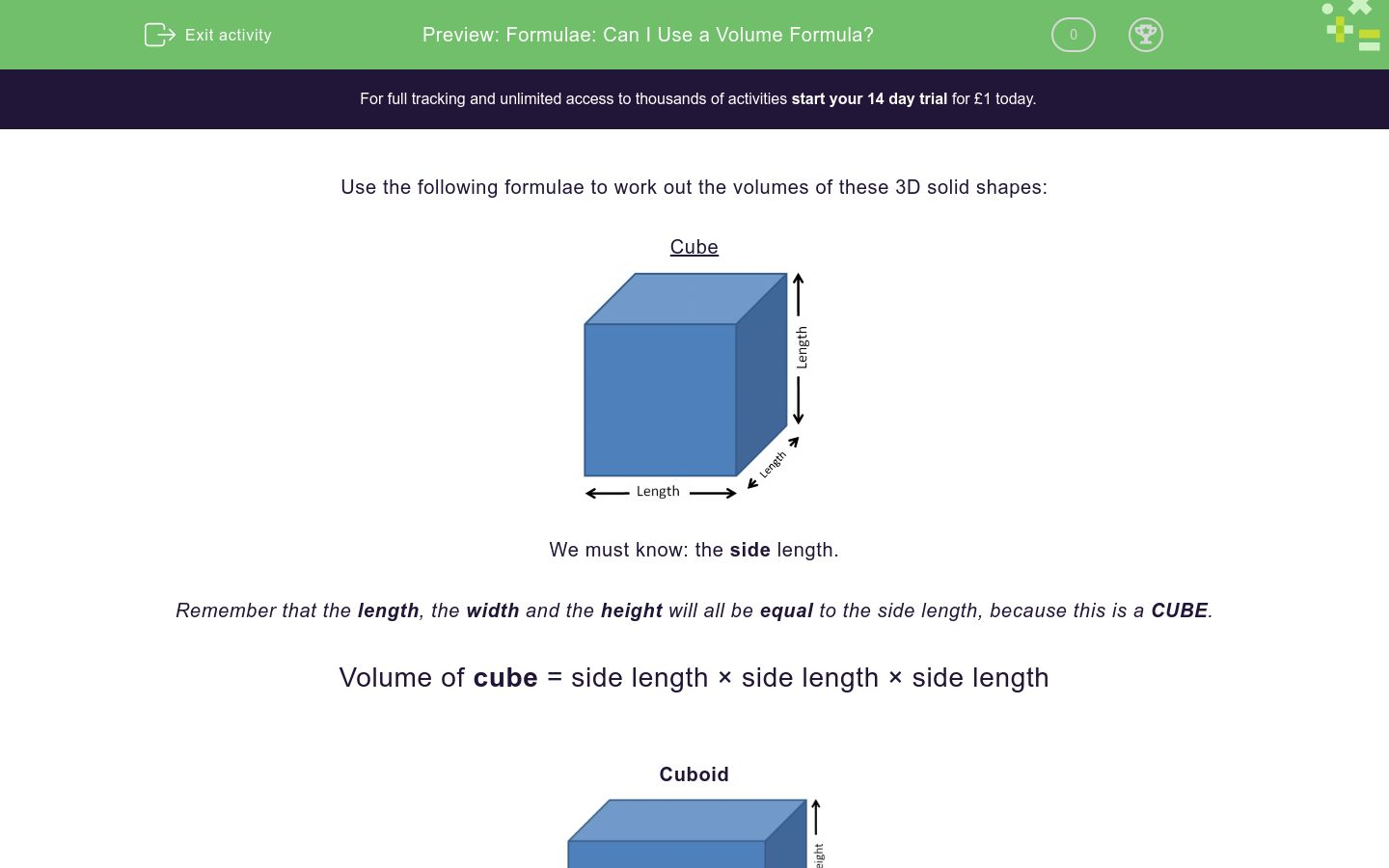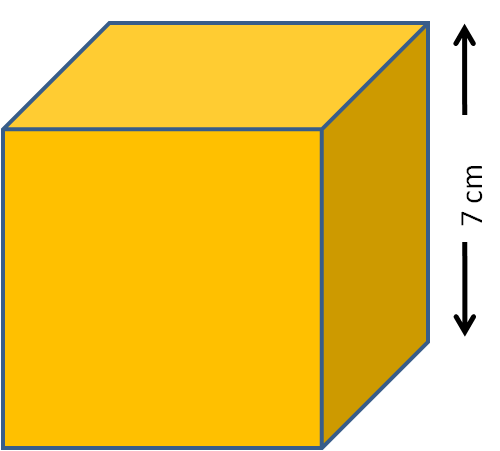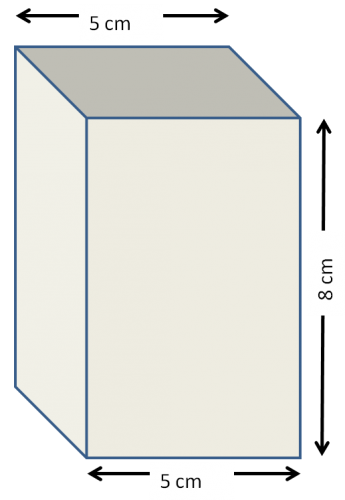# Formulae: Can I Use a Volume Formula?

In this worksheet, students must recognise when it is possible to use formulae for the volume of the given shapes.Key stage:  KS 2

Curriculum topic:   Measurement

Curriculum subtopic:   Use Area/Volume Formulae

Difficulty level:### QUESTION 1 of 10

Use the following formulae to work out the volumes of these 3D solid shapes:

CubeWe must know: the side length.

Remember that the length, the width and the height will all be equal to the side length, because this is a CUBE.

Volume of cube = side length × side length × side length

CuboidWe must know: the length; the width; and the height.

Volume of cuboid = length × width × height

e.g. Can a volume formula be used to work out the volume of this cuboid, and if so, which one is best?This is a cuboid.

We must know: the length; the width; and the height.

The length is 15 cm, the width is 4 cm and the height is 8 cm.

Yes, we can use the volume formula:

Volume of cuboid  = length × width × height

e.g. Can a volume formula be used to work out the volume of this cube, and if so, which one is best?This is a cube.

We must know: the side length.

The side length is 7 cm, so the width and the height are also 7 cm.

Yes, we can use the volume formula:

Volume of cube  = side length × side length × side length

e.g. Can a volume formula be used to work out the volume of this cuboid, and if so, which one is best?This is a cuboid.

We must know: the length; the width; and the height.

The length is 9 cm, the width is 4 cm but we do not know the height.

No, we cannot use a volume formula.

NB: The length, the width and the height are all interchangeable with each other, depending on how you look at a cuboid.

Want to understand this further and learn how this links to other topics in maths?

Why not watch this video?

Choose the best volume formula to be used to work out the volume of this cuboid.volume = side length × side length × side length

volume = length × width × height

not possible to work out volume

Choose the best volume formula to be used to work out the volume of this cube.volume = side length × side length × side length

volume = length × width × height

not possible to work out volume

Choose the best volume formula to be used to work out the volume of this cuboid.volume = side length × side length × side length

volume = length × width × height

not possible to work out volume

Choose the best volume formula to be used to work out the volume of this cuboid.volume = side length × side length × side length

volume = length × width × height

not possible to work out volume

Choose the best volume formula to be used to work out the volume of this cube.volume = side length × side length × side length

volume = length × width × height

not possible to work out volume

Choose the best volume formula to be used to work out the volume of this cuboid.volume = side length × side length × side length

volume = length × width × height

not possible to work out volume

Choose the best volume formula to be used to work out the volume of this cube.volume = side length × side length × side length

volume = length × width × height

not possible to work out volume

Choose the best volume formula to be used to work out the volume of this cuboid.volume = side length × side length × side length

volume = length × width × height

not possible to work out volume

Choose the best volume formula to be used to work out the volume of this cube.volume = side length × side length × side length

volume = length × width × height

not possible to work out volume

Choose the best volume formula to be used to work out the volume of this cuboid, which has a square end as shown.volume = side length × side length × side length

volume = length × width × height

not possible to work out volume

• Question 1

Choose the best volume formula to be used to work out the volume of this cuboid.volume = length × width × height
EDDIE SAYS
This is a cuboid.
We know the length, the width and the height.
• Question 2

Choose the best volume formula to be used to work out the volume of this cube.volume = side length × side length × side length
EDDIE SAYS
This is a cube.
All the side lengths are 5 cm.
• Question 3

Choose the best volume formula to be used to work out the volume of this cuboid.not possible to work out volume
EDDIE SAYS
This is a cuboid.
We know two of the length, the width and the height, but we need all three.
• Question 4

Choose the best volume formula to be used to work out the volume of this cuboid.not possible to work out volume
EDDIE SAYS
This is a cuboid.
The 5 cm is shown twice as the width.
We know two of the length, the width and the height, but we need all three.
• Question 5

Choose the best volume formula to be used to work out the volume of this cube.volume = side length × side length × side length
EDDIE SAYS
This is a cube.
All the side lengths are 5 mm.
• Question 6

Choose the best volume formula to be used to work out the volume of this cuboid.volume = length × width × height
EDDIE SAYS
This is a cuboid.
The 5 cm is shown twice as the width.
We know the length, the width and the height, so we can use the volume formula for a cuboid.
• Question 7

Choose the best volume formula to be used to work out the volume of this cube.volume = side length × side length × side length
EDDIE SAYS
This is a cube.
All the side lengths are 9 cm even though only two of them are shown.
• Question 8

Choose the best volume formula to be used to work out the volume of this cuboid.not possible to work out volume
EDDIE SAYS
This is a cuboid.
The 15 cm is shown three times as the length.
We do not know the width or the height.
• Question 9

Choose the best volume formula to be used to work out the volume of this cube.not possible to work out volume
EDDIE SAYS
This is a cube.
All the side lengths are the same but we have no information on these lengths.
• Question 10

Choose the best volume formula to be used to work out the volume of this cuboid, which has a square end as shown.volume = length × width × height
EDDIE SAYS
This is a cuboid.
The length is 20 cm.
The width is 9 cm as shown.
The height is also 9 cm because the end is a square.
---- OR ----

Sign up for a £1 trial so you can track and measure your child's progress on this activity.

### What is EdPlace?

We're your National Curriculum aligned online education content provider helping each child succeed in English, maths and science from year 1 to GCSE. With an EdPlace account you’ll be able to track and measure progress, helping each child achieve their best. We build confidence and attainment by personalising each child’s learning at a level that suits them.

Get started